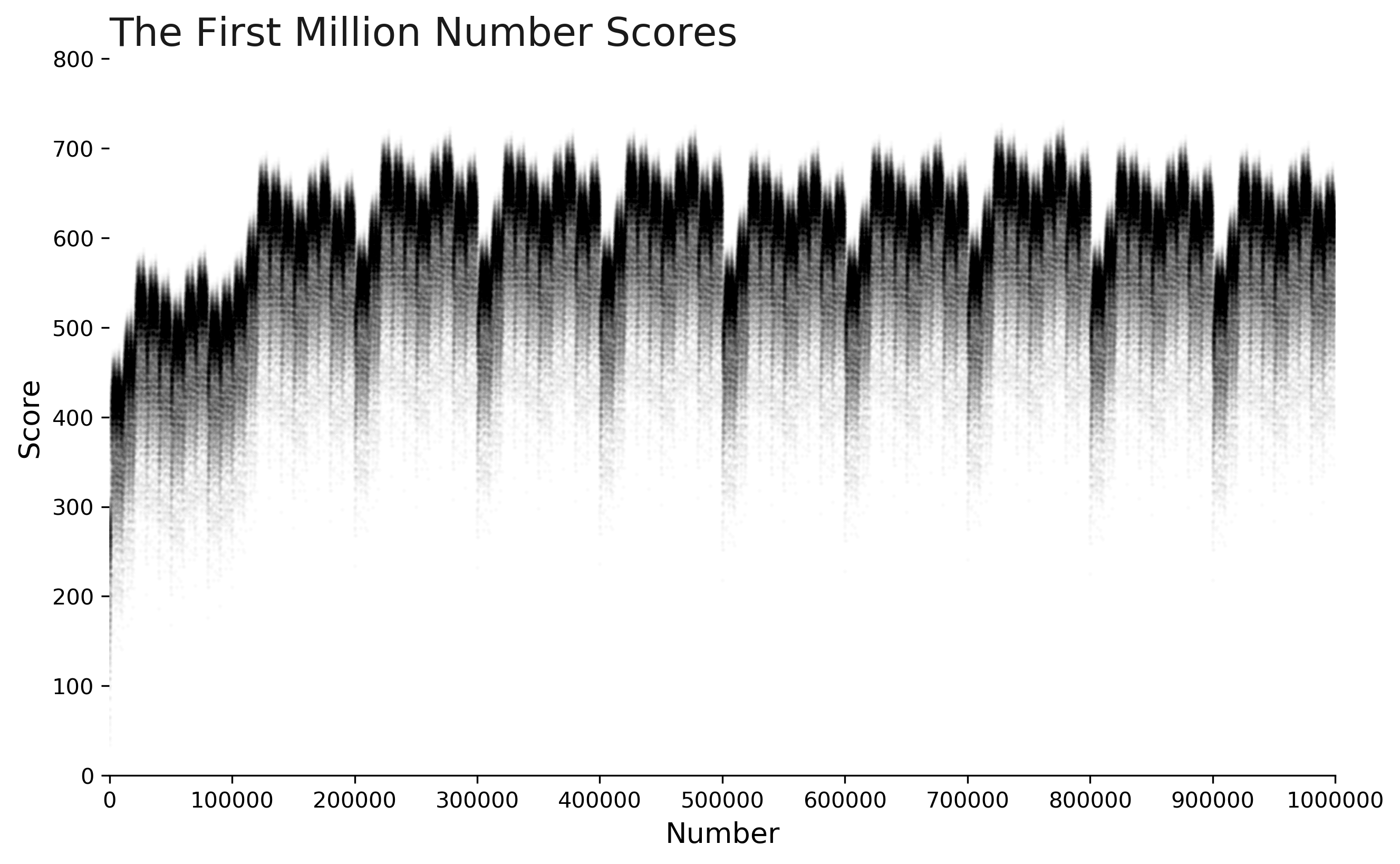Jan 10, 2020

# Introduction

Gematria is a numerical system that links Hebrew characters to numbers. This week's Riddler Classic explores the "score" of a number, and asks us to identify any patterns that emerge.

In Jewish study, “Gematria” is an alphanumeric code where words are assigned numerical values based on their letters. We can do the same in English, assigning 1 to the letter A, 2 to the letter B, and so on, up to 26 for the letter Z. The value of a word is then the sum of the values of its letters. For example, RIDDLER has an alphanumeric value of 70, since R + I + D + D + L + E + R becomes 18 + 9 + 4 + 4 + 12 + 5 + 18 = 70.

But what about the values of different numbers themselves, spelled out as words? The number 1 (ONE) has an alphanumeric value of 15 + 14 + 5 = 34, and 2 (TWO) has an alphanumeric value of 20 + 23 + 15 = 58. Both of these values are bigger than the numbers themselves.

Meanwhile, if we look at larger numbers, 1,417 (ONE THOUSAND FOUR HUNDRED SEVENTEEN) has an alphanumeric value of 379, while 3,140,275 (THREE MILLION ONE HUNDRED FORTY THOUSAND TWO HUNDRED SEVENTY FIVE) has an alphanumeric value of 718. These values are much smaller than the numbers themselves.

If we consider all the whole numbers that are less than their alphanumeric value, what is the largest of these numbers?

# Solution

The lucky number for this weeks solution is 279. With a score of 284, it is the largest integer that doesn't exceed its score.If we test the first million numbers we see clear patterns emerge (well, maybe not crystal clear because rendering a million points doesn't make for the sharpest image.) The cyclical shape tends to range between values of 500-700 for most of the numbers up to one million.# Full Code

Lots of static dictionaries and testing in this code, which make it appear longer than it really is. In reality, the two short class methods, Number.spell and Number.score are the heavy-lifters, and they run very quickly! It takes just less than one second to run the first hundred thousand numbers.

import doctest

class Number(int):

letter_score = " abcdefghijklmnopqrstuvwxyz"
letter_score = {k: v for v, k in enumerate(letter_score)}
spelling = {
0: "",
1: "one",
2: "two",
3: "three",
4: "four",
5: "five",
6: "six",
7: "seven",
8: "eight",
9: "nine",
10: "ten",
11: "eleven",
12: "twelve",
13: "thirteen",
14: "fourteen",
15: "fifteen",
16: "sixteen",
17: "seventeen",
18: "eighteen",
19: "nineteen",
20: "twenty",
30: "thirty",
40: "forty",
50: "fifty",
60: "sixty",
70: "seventy",
80: "eighty",
90: "ninety",
}

@classmethod
def spell(cls, n: int) -> str:
"""
Convert an integer into a spelled word, e.g. 42 -> forty two

Examples
--------
>>> spell = Number.spell
>>> spell(18)
'eighteen'
>>> spell(61)
'sixty one'
>>> spell(100)
'one hundred'
>>> spell(118)
'one hundred eighteen'
>>> spell(178)
'one hundred seventy eight'
>>> spell(999)
'nine hundred ninety nine'
>>> spell(10018)
'ten thousand eighteen'
>>> spell(78123)
'seventy eight thousand one hundred twenty three'
>>> spell(987654)
'nine hundred eighty seven thousand six hundred fifty four'
>>> spell(118406714)
'one hundred eighteen million four hundred six thousand seven hundred fourteen'
>>> spell(12000000012)
'twelve billion twelve'
>>> spell(1000000000000)
Traceback (most recent call last):
ValueError: Unable to convert numbers larger than 999,999,999,999
"""
try:
return cls.spelling[n]
except KeyError:
if n < 100:
return f"{cls.spell((n // 10) * 10)} {cls.spell(n % 10)}"
if n < 1e3:
return f"{cls.spell(n // 100)} hundred {cls.spell(n % 100)}".strip()
if n < 1e6:
return f"{cls.spell(n // 1e3)} thousand {cls.spell(n % 1e3)}".strip()
if n < 1e9:
return f"{cls.spell(n // 1e6)} million {cls.spell(n % 1e6)}".strip()
if n < 1e12:
return f"{cls.spell(n // 1e9)} billion {cls.spell(n % 1e9)}".strip()
raise ValueError("Unable to convert numbers larger than 999,999,999,999")

@classmethod
def score(cls, n: int) -> int:
"""
Returns the 'score' of an integer, where score is calculated by spelling
the word (e.g. 42 becomes 'forty two'), then assigning a value to each
of the characters (a=1, b=2, c=3, ..., z=26) and adding them up.

Examples
--------
>>> score = Number.score
>>> score(2)
58
>>> score(42)
142
>>> score(1417)
379
>>> score(3140275)
718
"""
return sum(cls.letter_score[letter] for letter in cls.spell(n))

if __name__ == "__main__":
doctest.testmod()

# sample generator for the scores of the first 100,000 integers
scores = (Number.score(x) for x in range(100000))